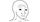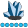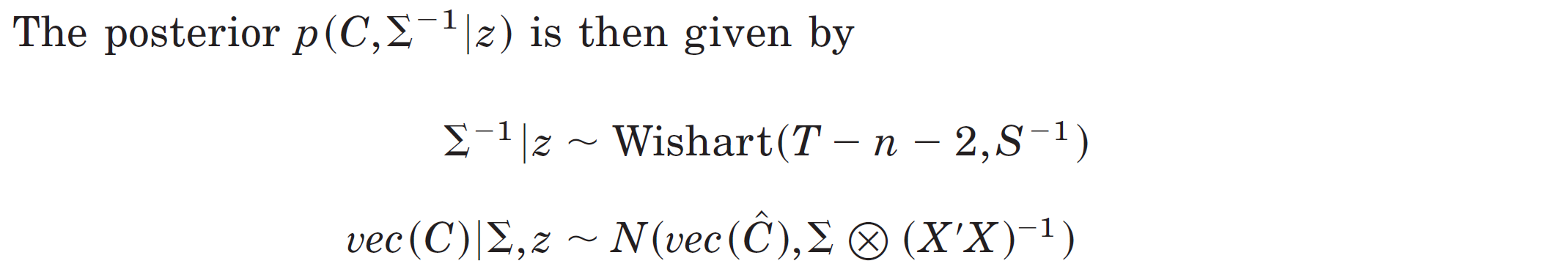somebody
Lapis Lazuli | Level 10

## draw samples from distribution whose parameters are matrices

Hi all,

I would like to draw samples from some distribution with parameters in matrix form. For example, a normal distribution N(mu,sigma) takes to parameters mu and sigma which are numbers. However, my mu and sigma are now matrices.  In particular, the distribution of interest is:where S is a 4x4 matrix, X is a 100x4 matrix.

Thanks,

1 ACCEPTED SOLUTION

Accepted Solutions

## Re: draw samples from distribution whose parameters are matrices

This seems to be crossposted to StackOverflow. Please only post in one place unless you are not getting a satisfactory response.  For completeness, here is the solution for data from the Sashelp.Cars data. The randomly generated C matrix is very close to the C_hat matrix for the data.

``````proc iml;
use sashelp.cars;
read all var {weight wheelbase enginesize horsepower} into X;
read all var {mpg_city mpg_highway} into Z;
close;

*normal equations and covariance;
xpx = x`*x;
invxpx = inv(xpx);
C_hat = invxpx*(x`*z);
r = z-x*c_hat;
S = r`*r;

*draw sigma;
call randseed(4321);
DF = nrow(X)-ncol(X)-2;
W = RandWishart(1, DF, inv(S));  /* 1 x (p*p) row vector */
sigma = shape(W, sqrt(ncol(W))); /* reshape to p x p matrix */
*draw vec(c);
vec_c_hat = colvec(c_hat`);      /* stack columns of c_hat */
vec_c = RandNormal(1, vec_c_hat, sigma@invxpx);
c = shapecol(vec_c, nrow(C_hat), ncol(C_hat));   /* reshape w/ SHAPECOL */
print C_hat, c;``````
4 REPLIES 4

## Re: draw samples from distribution whose parameters are matrices

The RANDNORMAL function in SAS/IML can handle this.

You can generate samples from the multivariate normal distribution in SAS following the article by Rick Wicklin here

http://blogs.sas.com/content/iml/2013/04/10/generate-multiple-mvn-samples.html

## Re: draw samples from distribution whose parameters are matrices

You can use the RANDWISHART function in SAS/IML to draw samples (matrices) from the Wishart distribution.

In addition to the documentation, you can read the article "The Wishart distribution: Covariance matrices for multivariate normal data," which provides a discussion and example as well as how to .reshape the output.

If necessary, you can also use the RANDNORMAL function to sample from a multivariate normal distribution.

## Re: draw samples from distribution whose parameters are matrices

This seems to be crossposted to StackOverflow. Please only post in one place unless you are not getting a satisfactory response.  For completeness, here is the solution for data from the Sashelp.Cars data. The randomly generated C matrix is very close to the C_hat matrix for the data.

``````proc iml;
use sashelp.cars;
read all var {weight wheelbase enginesize horsepower} into X;
read all var {mpg_city mpg_highway} into Z;
close;

*normal equations and covariance;
xpx = x`*x;
invxpx = inv(xpx);
C_hat = invxpx*(x`*z);
r = z-x*c_hat;
S = r`*r;

*draw sigma;
call randseed(4321);
DF = nrow(X)-ncol(X)-2;
W = RandWishart(1, DF, inv(S));  /* 1 x (p*p) row vector */
sigma = shape(W, sqrt(ncol(W))); /* reshape to p x p matrix */
*draw vec(c);
vec_c_hat = colvec(c_hat`);      /* stack columns of c_hat */
vec_c = RandNormal(1, vec_c_hat, sigma@invxpx);
c = shapecol(vec_c, nrow(C_hat), ncol(C_hat));   /* reshape w/ SHAPECOL */
print C_hat, c;``````somebody
Lapis Lazuli | Level 10

## Re: draw samples from distribution whose parameters are matrices

Thanks @Rick_SAS for pointing out my error in the Stackoverflow post.

From The DO Loop